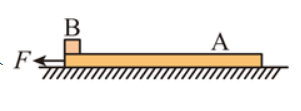(1) 长木板在加速过程中, 物体 $B$ 的加速度 $a_B$ 及水平外力 $F$ 的大小;
（2）长木板 $A$ 的长度 $L$ 。【解答】解: (1) 设物体 $B$ 的最大加速度为 $a_m$, 对 $B$ 根据牛顿第二定律可得:
$$a_m=\frac{\mu m g}{m}=\mu g=0.1 \times 10 m / \mathrm{s}^2=1 \mathrm{~m} / \mathrm{s}^2$$

$$F-\mu m g-\mu(m+M) g=M a$$

(2) 设长木板加速时间为 $t_1$, 通过的位移为 $x_1$, 在 $t_1$ 时刻 $B$ 物体获得的速度为 $v_1$, 则:
\begin{aligned} & t_1=\frac{v}{a}=\frac{6}{2}=3 \mathrm{~s} \\ & x_1=\frac{1}{2} a_t^2=\frac{1}{2} \times 2 \times 3^2 \mathrm{~m}=9 \mathrm{~m} \\ & v_1=a_m t_1=1 \times 3 \mathrm{~m} / \mathrm{s}=3 \mathrm{~m} / \mathrm{s} \end{aligned}

$$\mu m g+\mu(M+m) g=M a_A$$

$B$ 在这两个过程中一直都是匀加速直线运动, 其位移为:
$$x_B=\frac{1}{2} a_B\left(t_1+t_2\right)^2$$

( 2 ） 长木板 $A$ 的长度为 $6 m$ 。